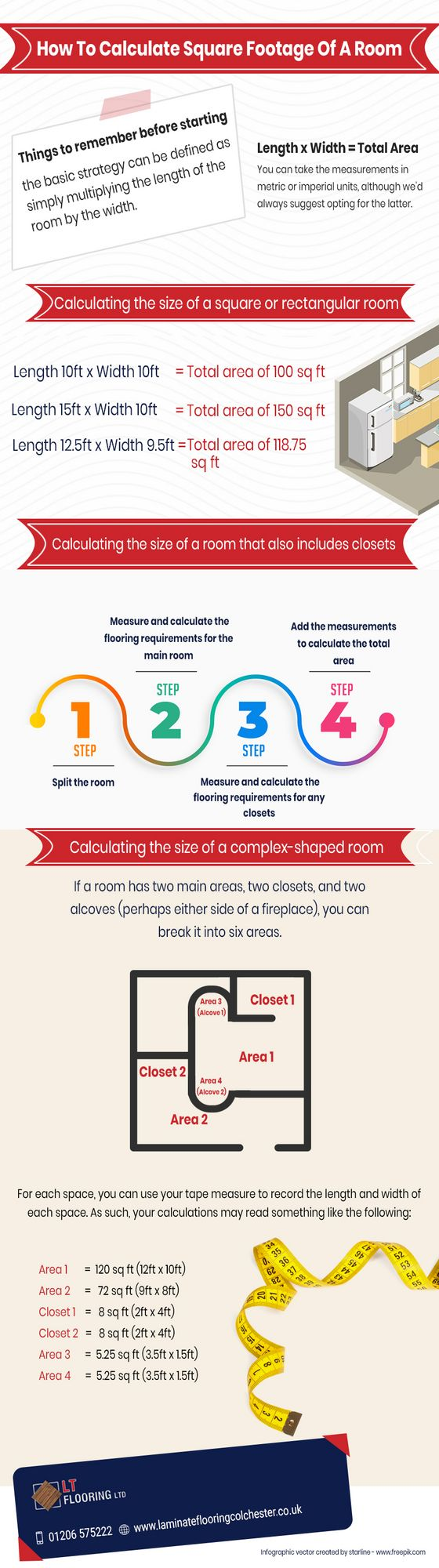Posted: December 17, 2019
Submitted by: https://laminateflooringcolchester.co.uk/

How To Calculate Square Footage Of A Room

If you are planning to upgrade your home interior with new flooring and furniture, it is easy to figure out your square footage.

The basic strategy can be defined as simply multiplying the length of the room by the width.

Length x Width = Total Area

### How To Calculate Square Footage Of A Room

For calculating the square footage of a room that is a singular rectangle or square shape, you need to measure the length of the room from wall-to-wall and the width of the room from wall-to-wall,

Here are some examples:

1) Length 10ft x Width 10ft = Total area of 100 sq ft

2) Length 15ft x Width 10ft = Total area of 150 sq ft

3) Length 12.5ft x Width 9.5ft = Total area of 118.75 sq ft

It does take time to plan a new flooring project. To start, you will need:

1) Tape measure

2) Calculator

Original Post: How To Calculate Square Footage Of A Room

Categories:

Open Infographic Image In New Tab

Embed this infographic:

`<a href="https://infographicsite.com/infographic/how-to-calculate-square-footage-of-a-room/"><img src="https://infographicsite.com/wp-content/uploads/2019/12/laminate-flooring.jpg" alt="How To Calculate Square Footage Of A Room" /></a>`

Is there a problem with this infographic? Please let us know!